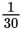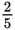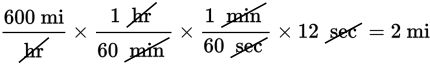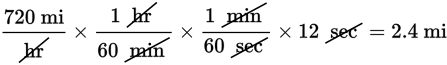# SAT Math Multiple Choice Question 503: Answer and Explanation

### Test Information

Question: 503

8. An airline company purchased two new airplanes. One can travel at speeds of up to 600 miles per hour and the other at speeds of up to 720 miles per hour. How many more miles can the faster airplane travel in 12 seconds than the slower airplane?

• A.• B.• C. 2
• D. 30

Explanation:

B

Difficulty: Medium

Category: Problem Solving and Data Analysis / Rates, Ratios, Proportions, and Percentages

Strategic Advice: Let the units in this question guide you to the solution. The speeds of the airplanes are given in miles per hour, but the question asks about the number of miles each airplane can travel in 12 seconds, so convert miles per hour to miles per second.The faster plane can travel 2.4 – 2 = 0.4 miles farther, which is the same asmiles.